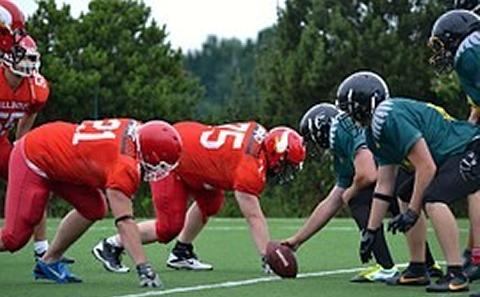The University of Southampton
Mathematical Sciences

# Research Group: Game Theory

Currently Active:
Yes

Game theory is a mathematical discipline that studies settings involving multiple decision makers (players). Two major branches of game theory are cooperative game theory and non-cooperative game theory, depending on whether players are collaborating or competing against each other. In cooperative game theory, players can form coalitions to jointly achieve some objectives which are associated by some payoff values (representing the costs/profits). Our research group focuses largely on how payoffs are shared among players in a fair and stable way and on how coalitions are formed. We develop computational optimisation techniques for computing solutions of large games, and also study stochastic games in which the characteristic function is uncertain. We are also interested in Stackelberg games, that is, in games where the players take turns according to a hierarchy, and in the bilevel programming aspect that the corresponding equilibrium finding problem entails. Recent applications include supply chain coordination, multi-agent systems optimisation in finance, and the computation of leader-follower Nash equilibria.## Coalition Structure Formation

The first problem on coalition structure formation has recently received a lot of attention from the algorithmic game theory community and the artificial intelligence community. This problem is equivalent to a set covering problem in the operations research literature. The main aim of this project is to exploit the special property of the characteristic functions and develop mathematical programming techniques to solve the problem more efficiently.## Equilibrium finding algorithms

In spite of the vast richness of solution concepts that non-cooperative game theory presents, computational methods capable of constructing one such solution either exactly or within a given precision are, in many cases, lacking. In this project, we aim at developing computationally tractable algorithms to compute different definition of equilibria arising in various type of games. Among others, we mention the computation of leader-follower Nash equilibria for Stackelberg games where, after the leader's commitment, the followers play, simultaneously, a Nash equilibrium, and the computation of so-called team max-min equilibria. We mainly employ mixed-integer (nonlinear) and bilevel programming techniques, as well as those of combinatorial optimization.## Optimization Methods for Cooperative Games

Although there is an extensive literature on the solution properties of cooperative games, computing them is still very challenging due to their combinatorial structures. One of the objective of this project is to develop mathematical models for finding these solutions. These models will be large-scale optimisation problems because of their combinatorial nature. For example, finding the least core can be formulated as a large linear programming problem while finding the nucleolus involves solving nested linear programs.